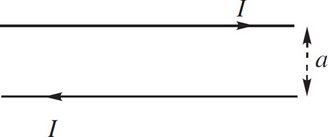# AP Physics 2 Practice Test 3

### Test Information10 questions18 minutes

1. A capacitor C is connected to a resistor Q in series. If the capacitor's initial charge was Q, what is the average power dissipated through the resistor if it loses half of its charge in a time t ?

2. If L, M, and T denote the dimensions of length, mass, and time, respectively, what are the dimensions of power?

3. Consider two adjacent transparent media. The speed of light in Medium 1 is v1, and the speed of light in Medium 2 is v2. If v1 < v2, then total internal reflection will occur at the interface between these media if a beam of light is

4. Traveling at an initial speed of 1.5 × 106 m/s, a proton enters a region of constant magnetic field of magnitude 1.5 T. If the proton's initial velocity vector makes an angle of 30° with the magnetic field, compute the proton's speed 4 s after entering the magnetic field.

5. A photocell of work function ϕ = 2eV is connected to a resistor in series. Light of frequency f = 1 × 1015 Hz hits a metal plate of the photocell. If the power of the light is P = 100 W, what is the current through the resistor?

6. An electric dipole consists of a pair of equal but opposite point charges of magnitude 4.0 nC separated by a distance of 2.0 cm. What is the electric field strength at the point midway between the charges?

7. In an experiment designed to study the photoelectric effect, it is observed that low-intensity visible light of wavelength 550 nm produced no photoelectrons. Which of the following best describes what would occur if the intensity of this light were increased dramatically?

8.Electric current is established in each of two long parallel wires, as shown in the figure above. Describe the force per unit length on each wire.

9. An object is placed 100 cm from a plane mirror. How far is the image from the object?

10. An ideal fluid flows through a pipe with radius Q and flow speed V. If the pipe splits up into three separate paths, each with radius (Q/2), what is the flow speed through each of the paths?